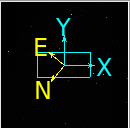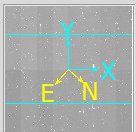# Questions related to the method to_boresight_rotation_angle and config.detectorList[i].yawDeg in camera.py

Recently I am dealing with my image and I met some problems with my rotated image, the wcs of most of my CCD is:``````CD1_1   =   -6.79797745287E-05 / Transformation matrix
CD1_2   =    6.24916233727E-05 / no comment
CD2_1   =   -6.25091917885E-05 / no comment
CD2_2   =   -6.79390630166E-05 / no comment
INR-STR =    137.3915692167144
``````

and my method to_boresight_rotation_angle is

``````def to_boresight_rotation_angle(self):
angle = Angle(self.quantity_from_card("INR-STR", u.deg))
angle = angle.wrap_at("360d")
return angle
``````

After test, I find in your pipeline, counterclockwise is the positive direction, so you can see in the above I set

``````INR-STR =    137.3915692167144
``````

and I can deal with these image properly, however, if I switch to the image that have different yawDeg, for example, these CCDs have the following wcs:``````CD1_1   =   -6.17345939303E-05 / Transformation matrix
CD1_2   =   -6.86453518046E-05 / no comment
CD2_1   =    6.86872826288E-05 / no comment
CD2_2   =   -6.16748668907E-05 / no comment
(INR-STR is the same)
INR-STR =    137.3915692167144
``````

and you can see, the rotation angle of these CCDs is 90 deg larger thnn before, to deal with the rotation, I set the yaw deg

``````config.detectorList[i].yawDeg = 90.0
``````

but this time, I can’t process these CCDs properly, and I check up the wcs the pipeline get by:

``````butler = Butler(butler_path)
registry = butler.registry
raw_wfst = butler.get('raw',visit=1,instrument='WFST',detector=3,exposure=1,collections='WFST/raw/all')
wcs = raw_wfst.wcs
wcs.getCdMatrix()
``````

some information in my camera.py related to wcs:

``````config.transformDict.transforms['FieldAngle'].transform['affine'].linear = [-0.3324/2062.65, 0.0, 0.0, 0.3324/2062.65]
``````

and for the CCDs without rotation, the wcs in the pipeline is correct, yaw=0:

``````array([[-6.74663723e-05,  6.20568336e-05],
[-6.20568336e-05, -6.74663723e-05]])
``````

but for the CCDs without rotation, things went wrong, yaw=90, but the wcs I get is the same as before,

``````array([[-6.85898470e-05,  6.16470209e-05],
[-6.16470209e-05, -6.85898470e-05]])
``````

but if I try with some simulated data, the yaw deg work, for example:
yaw =0

``````array([[9.23332466e-05, 0.00000000e+00],
[0.00000000e+00, 9.23332466e-05]])
``````

yaw = 90

``````array([[ 5.65378075e-21, -9.23332466e-05],
[ 9.23332466e-05,  5.65378075e-21]])
``````

and the match is fail undoubtly, so what may cause this, is it because the total rotation angle is greater than 180? but there are many 270 in hsc’s camera.py, and what’s the standard about the to_boresight_rotation_angle and config.detectorList[i].yawDeg in your system?

Thank you!

CD matrices do contain rotation information (and you can see the signs of the CD matrix have changed in the second example) but I’m confused that the INR-STR has the same value. Are you saying your chips have different orientations in the focal plane? HSC does have some flipped detectors and we fix their WCS on read:

where the corners have a different formatter so have different orientations.

If you want to check your code you can call